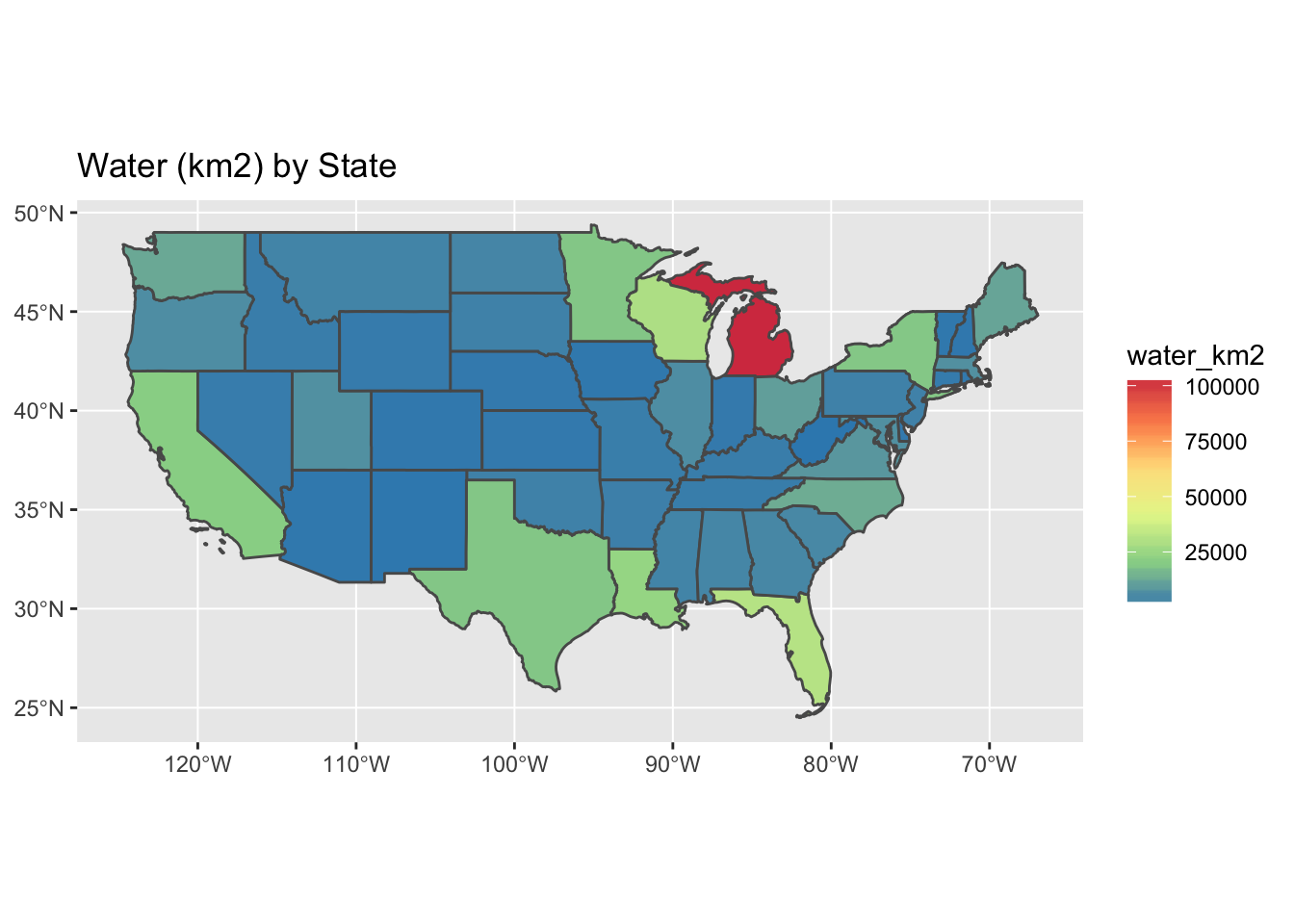# Lesson 2 Interactive Maps

## 2.1 Overview

Questions

• How do you generate interactive plots of spatial data to enable pan, zoom and hover/click for more detail?

Objectives

Learn variety of methods for producing interactive spatial output using libraries:

• `plotly`: makes any ggplot2 object interactive
• `mapview`: quick view of any spatial object
• `leaflet`: full control over interactive map

## 2.2 Things You’ll Need to Complete this Tutorial

R Skill Level: Intermediate - you’ve got basics of R down.

We will continue to use the `sf` and `raster` packages and introduce the `plotly`, `mapview`, and `leaflet` packages in this tutorial.

``````# load packages
library(tidyverse)  # loads dplyr, tidyr, ggplot2 packages
library(sf)         # simple features package - vector
library(raster)     # raster
library(plotly)     # makes ggplot objects interactive
library(mapview)    # quick interactive viewing of spatial objects
library(leaflet)    # interactive maps

# set working directory to data folder
# setwd("pathToDirHere")``````

## 2.3 States: ggplot2

Recreate the ggplot object from Lesson 1 and save into a variable for subsequent use with the `plotly` package.

``````# read in states
st_zm() %>%
mutate(
water_km2 = (AWATER / (1000*1000)) %>% round(2))

# plot, ggplot
g = ggplot(states) +
geom_sf(aes(fill = water_km2)) +
scale_fill_distiller("water_km2", palette = "Spectral") +
ggtitle("Water (km2) by State")
g``````## 2.4 States: plotly

The `plotly::ggplotly()` function outputs a ggplot into an interactive window capable of pan, zoom and identify.

``````library(plotly)

ggplotly(g)``````

## 2.5 States: mapview

The `mapview::mapview()` function can work for a quick view of the data, providing choropleths, background maps and attribute popups. Performance varies on the object and customization can be tricky.

``````library(mapview)

# simple view with popups
mapview(states)``````
``````# coloring and layering
mapview(states, zcol='water_km2', burst='STUSPS')``````